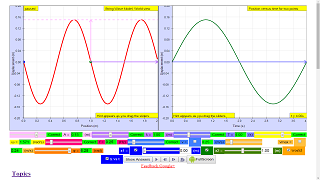## Topics

Progressive waves
Transverse and longitudinal waves
Determination of frequency and wavelength
Stationary waves

## Description

In this Wave representations simulation, you have two representations of wave motion to work with. The left one shows a movie of the wave traveling along a string - you can think of this representation as a sequence of photographs. The second right representation is a plot of the displacement as a function of time for two points on the string (you can select which two points to use). Using only these two representations, you can determine the values of many different parameters that describe the wave by changing the slider values or input fields. Hints have also been designed in for ease of visualizing the meaning of these parameters.

Play with the Wave Representations Model. Test what you've learned by exploring the amplitudes, wavelengths, periods, angular frequency, frequencies,  wave velocities, maximum transverse velocity (linking to simple harmonic motion) and phase difference.

## Sample Learning Goals

(a) show an understanding and use the terms displacement, amplitude, phase difference, period,
frequency, wavelength and speed
(b) deduce, from the definitions of speed, frequency and wavelength, the equation v = fλ
(c) recall and use the equation v = fλ
(f) analyse and interpret graphical representations of transverse and longitudinal waves
(c) SHM: understand and use the terms amplitude, period, frequency, angular frequency and phase difference and express the period in terms of both frequency and angular frequency

## Activities

Reset the simulation to get a new random wave you are happy with.
Amplitude, A and wavelength, λ  can be determined using the left graph of displacement versus position.
Then, play the simulation until you have a nice position versus time graph. Period, T and angular frequency, ω and frequency, f and phase difference between x1 and x2,  φ using the relationship φ/(2π) = t/T, where t is the time difference between x1 and x2, can be determined using the right graph of displacement of x1 and x2 versus time.

Using both graphs, it is possible to determine wave velocity v = f λ, maximum transverse speed of a single point on the string, vmax=  A ω. You can check your answers as many times as you want. The amplitude. The wavelength. The period. The angular frequency. The wave speed. The maximum transverse speed of a single point on the string.

## Version:

Wave representations

# Wave representations

In this simulation, you have two representations of wave motion to work with. One shows a movie of the wave traveling along a string - you can think of this representation as a sequence of photographs. The second representation is a plot of the displacement as a function of time for two points on the string (you can select which two points to use). Using only these two representations, you can determine the values of many different parameters that describe the wave.

Activities

# Keep pressing the "Get a new wave" button until you have a wave you are happy with. Then, play the simulation until you have a nice position versus time graph. Then, pause the simulation so that you have a photograph of the string as well as your position vs. time graph to work with. For each parameter below, figure out which of the two representations you need to determine the value of the parameter, and then find the value. You can check your answers as many times as you want.

1. The amplitude.
2. The wavelength.
3. The period.
4. The angular frequency.
5. The wave speed.
6. The maximum transverse speed of a single point on the string.

### Translations

Code Language Translator Run### Software Requirements

SoftwareRequirements

 Android iOS Windows MacOS with best with Chrome Chrome Chrome Chrome support full-screen? Yes. Chrome/Opera No. Firefox/ Samsung Internet Not yet Yes Yes cannot work on some mobile browser that don't understand JavaScript such as..... cannot work on Internet Explorer 9 and below

### Credits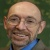Andrew Duffy; lookang; tina

### end faq

http://iwant2study.org/lookangejss/04waves_12generalwaves/ejss_model_Wave_representations_v5/Wave_representations_v5_Simulation.xhtml

## Apps

### Description

Introduction
Wave is an oscillation accompanied by a transfer of energy that travels through medium (space or mass)
Waves consist, instead, of oscillations or vibrations (of a physical quantity), around almost fixed locations
This simulation is on transverse wave where disturbance creates oscillations that are perpendicular to the propagation of energy transfer.
The equation for wave is A sin ( wt +kx + ϕ)
where
A is the maximum amplitude of the wave, maximum distance from the highest point of the disturbance in the medium (the crest) to the equilibrium point during one wave cycle.
w is is the angular frequency
t is time
k is is the wavenumber
x is the position
ϕ is the is the phase constant

concepts illustrated in the simulations include
T is the time for one complete cycle of an oscillation of a wave
f is the number of periods per unit time (per second) and is related by T = 1/f
λ is the wavelength
v is the velocity of the wave travelling and is related by v= f λ
vtmax is the maximum transverse velocity of the wave particle that occurs at the displacement d =0.

### Examples

EJSS wave representation model

Equation used to model the wave is $$y = A sin ( \omega t - k x )$$

## Amplitude A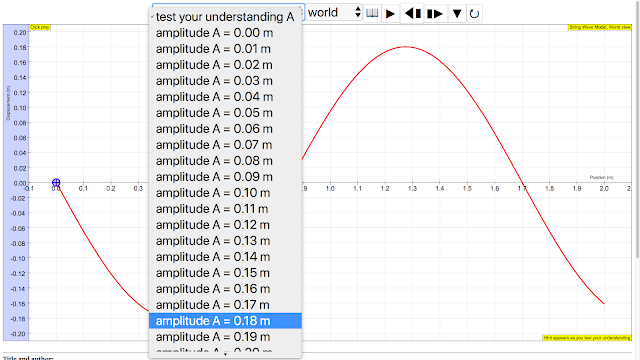amplitude of wave = 0.18 m, maximum displacement from equilibrium positionhttp://iwant2study.org/ospsg/index.php/112Direct Link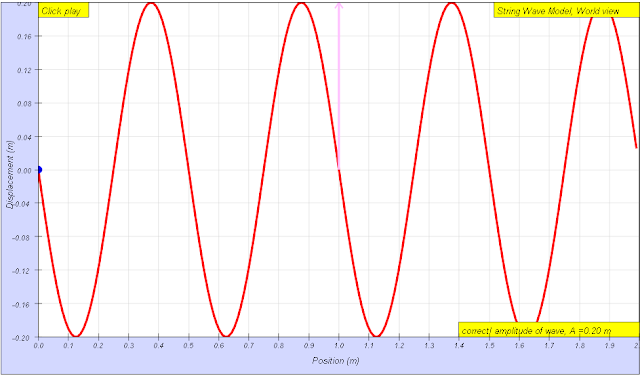amplitude of wave = 0.20 m, maximum displacement from equilibrium position run: Link1, Link2 download: Link1,source:  author: Andrew Duffy, lookang, tina author EJS: Francisco Esquembre

## wavelength λwavelength of wave = 1.70 m The wavelength λ  wavelength is the distance between two sequential crests or troughs (or other equivalent points)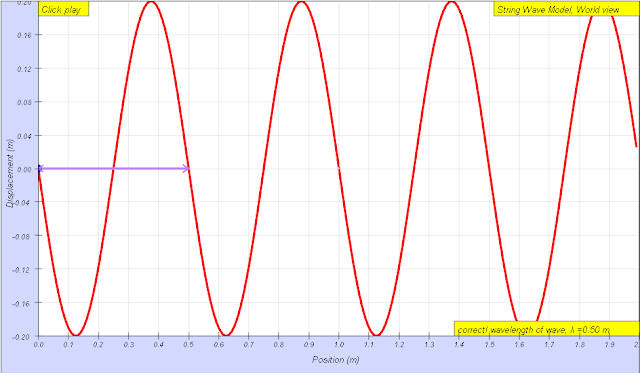wavelength of wave = 0.50 m The wavelength λ  wavelength is the distance between two sequential crests or troughs (or other equivalent points) run: Link1, Link2 download: Link1, Link2source:  author: Andrew Duffy, lookang, tina author EJS: Francisco Esquembre

## Period Tperiod of wave = 3.00 s, The period T is the time for one complete cycle of an oscillation of a wavehttp://iwant2study.org/ospsg/index.php/112Direct Linkperiod of wave = 3.00 s, The period T is the time for one complete cycle of an oscillation of a wave run: Link1, Link2 download: Link1, Link2source:  author: Andrew Duffy, lookang, tina author EJS: Francisco Esquembre

## angular frequency fThe frequency f = 1/T  is the number of periods per unit time (per second) and is typically measured in hertzhttp://iwant2study.org/ospsg/index.php/112Direct LinkThe frequency f = 1/T is the number of periods per unit time (per second) and is typically measured in hertz run: Link1, Link2 download: Link1, Link2source:  author: Andrew Duffy, lookang, tina author EJS: Francisco Esquembre

## angular velocity of wave ωangular frequencyω  = 2.09 s, The angular frequencyω represents the frequency in radians per second. run: Link1, Link2 download: Link1, Link2source:  author: Andrew Duffy, lookang, tina author EJS: Francisco Esquembre

## phase difference ϕthe phase of a vibration (that is, its position within the vibration cycle) ϕ measured in radians taking ratio "ϕ/(2π)= Δt/T",  ϕ = 2π(2.5-0.75)/3 =3.7 rad approximately

## wave speed v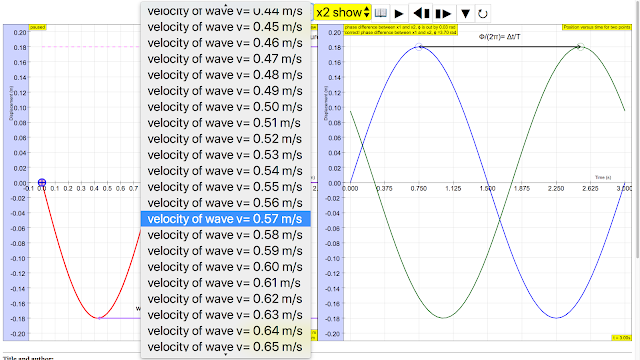sinusoidal waveform traveling at constant speed v is given by v = fsinusoidal waveform traveling at constant speed v is given by v = f λ run: Link1, Link2 download: Link1, Link2source:  author: Andrew Duffy, lookang, tina author EJS: Francisco Esquembre

## maximum transverse speed vmaxThe maximum transverse velocity is vmax = Aω and it occurs when the particle on the wave travels passes through the equilibrium positionhttp://iwant2study.org/ospsg/index.php/112Direct LinkThe maximum transverse velocity is vmax = Aω and it occurs when the particle on the wave travels passes through the equilibrium position run: Link1, Link2 download: Link1, Link2source:  author: Andrew Duffy, lookang, tina author EJS: Francisco Esquembre

## reference:

http://weelookang.blogspot.sg/2011/04/ejs-open-source-wave-representations.html

### Versions

1. http://weelookang.blogspot.sg/2015/08/ejss-wave-representation-model.html Blog post of JavaScript version of Wave Representations by Andrew Duffy and Loo Kang Wee
2. http://weelookang.blogspot.sg/2011/04/ejs-open-source-wave-representations.html Blog post of Java version of Wave Representations by Andrew Duffy and Loo Kang Wee
3. http://iwant2study.org/lookangejss/04waves_12generalwaves/ejs/ejs_model_Wave_representations_v5.jar Java version of Wave Representations by Andrew Duffy and Loo Kang Wee

### Other Resources

1. http://physics.bu.edu/~duffy/HTML5/wave_movie_and_graph.htmlby Andrew Duffy
2. http://www.phy.ntnu.edu.tw/ntnujava/index.php?topic=2405.0 One dimensional moving wave y(x,t)=A sin(k*x-w*t) by  Fu-Kwun Hwang